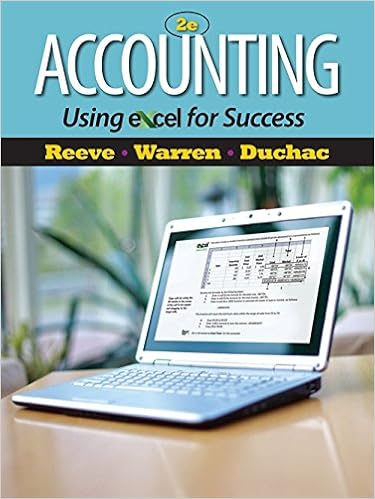# 101 102 103 104 105 the following information is

• Notes
• MajorFreedomCamel9406
• 41
• 100% (13) 13 out of 13 people found this document helpful

This preview shows page 16 - 22 out of 41 pages.

##### We have textbook solutions for you!
The document you are viewing contains questions related to this textbook.The document you are viewing contains questions related to this textbook.
Chapter 7 / Exercise 01
Accounting Using Excel for Success
Reeve/WarrenExpert Verified
101.
##### We have textbook solutions for you!
The document you are viewing contains questions related to this textbook.The document you are viewing contains questions related to this textbook.
Chapter 7 / Exercise 01
Accounting Using Excel for Success
Reeve/WarrenExpert Verified
102. 103. 104. 105. The following information is taken from the accounting records of Madeline Inc. for the year 2011. Missing information has been left blank. Inventory is the only supply that Madeline purchases on credit. Required: Compute the missing amounts.
106. 107. 108. 109.
110. Shown below is activity for one of the products of Denver Office Equipment: 111. Required: Compute the ending inventory and cost of goods sold assuming Denver uses FIFO. 112. Required: Compute the ending inventory and cost of goods sold assuming Denver uses LIFO and a perpetual inventory system. 113. Required: Compute the ending inventory and cost of goods sold assuming Denver uses average cost and a periodic inventory system. 114. Required: Compute the ending inventory and cost of goods sold assuming Denver uses average cost and a perpetual inventory system.
115. Required: Compute the ending inventory and cost of goods sold assuming Denver uses LIFO and a periodic inventory system. Shown below is the activity for one of the products of Random Creations: January 1 balance, 80 units @ \$50 \$4,000 116. Required: Compute the ending inventory and cost of goods sold assuming Random Creations uses FIFO. 117. Required: Compute the ending inventory and cost of goods sold assuming Random Creations uses LIFO and perpetual inventory system. 118. Required: Compute the ending inventory and cost of goods sold assuming Random Creations uses LIFO and a periodic inventory system. 119. Required: Compute the ending inventory and cost of goods sold assuming Random Creations uses average cost and a periodic inventory system.
120. Required: Compute the ending inventory and cost of goods sold assuming Random Creations uses average cost and a perpetual inventory system. 121.1. Calculate cost of goods sold for 2011. 2. Determine the amount of LIFO liquidation profit that the company must report in a disclosure note to its 2011 financial statements, assuming the amount is material. Assume an income tax rate of 40%. Bettencourt Clothing Corporation uses a periodic inventory system and the LIFO cost method. The company began 2011 with the following inventory layers (listed in chronological order of acquisition): During 2011, 20,000 units were purchased for \$15 per unit. Sales for the year totaled 30,000 units at various prices, leaving 3,000 units in ending inventory. Required: 122.Modern Day Appliances, Inc. is a wholesaler of kitchen appliances. The company uses a periodic inventory system and the LIFO cost method. Modern Day's December 31, 2011, fiscal year-end inventory of its main product, double-door, stainless steel refrigerators, consisted of the following (listed in chronological order of acquisition): The replacement cost of the refrigerators throughout 2012 was \$900. Modern Day sold 5,000 of these refrigerators during 2012. The company's selling price throughout 2012 was \$1,200. Required: 1. Compute the gross profit (sales minus cost of goods sold) and the gross profit ratio for 2012 assuming that Modern Day purchased 5,200 units during the year. 2. Repeat requirement 1 assuming that Modern Day purchased only 4,500 units.
•••